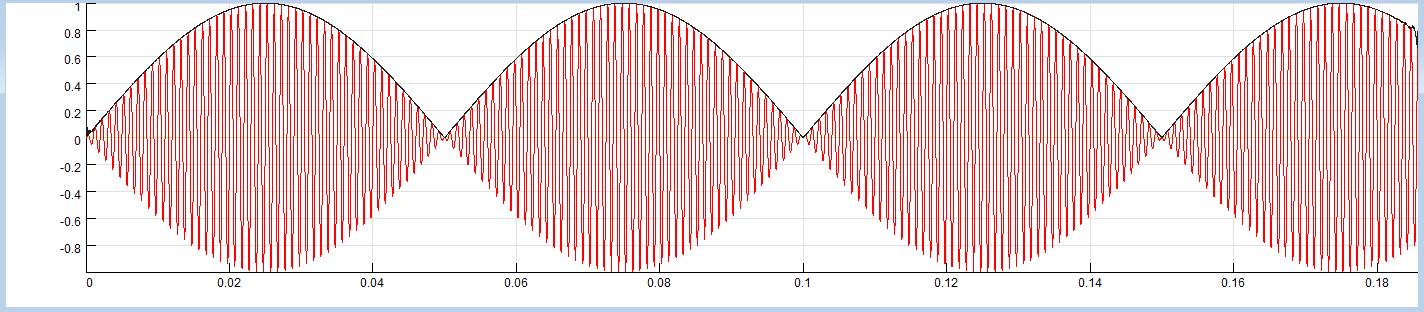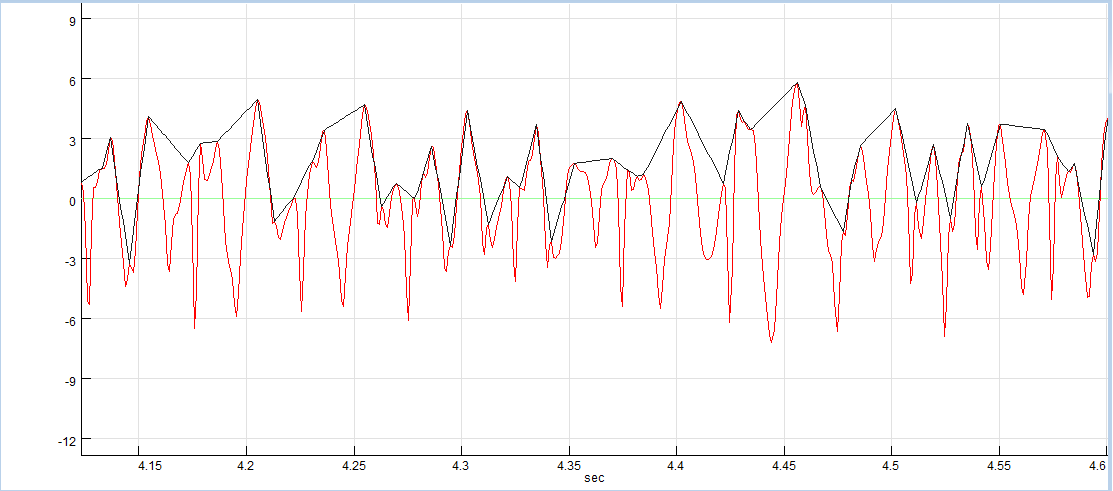Envelope is a smooth curve outlining a signal, for example by tracing signal peaks.

SIGVIEW offers two Julia-based custom tools for calculating envelope of a signal:

Hilbert transform method

This method uses Hilbert function and calculates the envelope as a magnitude of the analytic signal computed by using Julia's hilbert function. This method works well for signals with a clear dominant frequency (i.e. sine-like signals). For more complex signals with a lot of noise, you can try the "localmax" method.

The following example shows the original signal (red) with an overlay (black) calculated by using this tool.Localmax method

This method calculates the envelope signal by detecting local maximum values from a signal and connecting those with lines. It works better for real-world signals with a lot of noise.

The following example shows the original signal (red) with an overlay (black) calculated by using this tool.